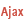HelpWLanguageWLanguage functionsControls, pages and windowsDrawing functionsPrefix syntaxDrawing functions on picLayer variablesDrawPolylinePresentationExampleCoordinatesDrawing in PHPDrawing in Browser codeRelated examplesSee alsoAlphaBlendBackgroundBlurChangeModeDrawArcDrawChordDrawCircleDrawLineDrawPointDrawPolygonDrawPolylineDrawRectangleDrawRectangleGradientDrawRoundedRectangleDrawSliceDrawTextDrawTextRTFEndDrawingFillFontHorizontalSymmetryInvertColorPenPixelColorPixelOpacityResizeRotationStartDrawingVerticalSymmetry
• Coordinates
• Drawing in PHP
• Drawing in Browser code
WINDEVWEBDEVWINDEV MobileOthers<picLayer variable>.DrawPolyline (Function)
In french: <Variable picCalque>.DessinePolyligneNot available
Draws a line composed of multiple segments in a variable of type picLayer.
The first coordinate indicates the starting point of the first segment, then each coordinate indicates the end of the previous segment and the beginning of the next segment.
Example
MyWDPicImage is WDPic = "Test.gif"
MyLayer is picLayer

MyLayer.Pen(LightBlue, 2, 4)
arrCoordinatesXY2 is array of 0 int = [2, 2, 140, 2, 150, 70, 0, 100, 200,100]
MyLayer.DrawPolyline(arrCoordinatesXY2)

IMG_MyDrawing = MyWDPicImage
Syntax

Building a line point by point

<picLayer image>.DrawPolyline(<NbPoint> , <X1> , <Y1> , <X2> , <Y2> [, <Xn> [, <Yn> [, <Line color>]]])
<picLayer image>: picLayer variable
Name of the picLayer variable to be used.
<NbPoint>: Integer
Number of line segments. This parameter defines the number of <X>, <Y> pairs to specify in the function.
<X1>: Integer
X-coordinate of the first segment of the line. These coordinates are expressed in pixels.
<Y1>: Integer
Y-coordinate of the first segment of the line. These coordinates are expressed in pixels.
<X2>: Integer
X-coordinate of the second segment of the line. These coordinates are expressed in pixels.
<Y2>: Integer
Y-coordinate of the second segment of the line. These coordinates are expressed in pixels.
<Xn>: Optional integer
X-coordinate of the N segment of the line. These coordinates are expressed in pixels.
<Yn>: Optional integer
Y-coordinate of the N segment of the line. These coordinates are expressed in pixels.
<Line color>: Integer or constant (optional)
Line color. This color can correspond to:
If this parameter is not specified, the line color:
• corresponds to the color specified in the last call to <Image>.Pen,
• is the same as the background color if <Image>.Pen has not been used.

Building a line via an array of X and Y-coordinates

<picLayer image>.DrawPolyline(<Array of coordinates> [, <Line color>])
<picLayer image>: picLayer variable
Name of the picLayer variable to be used.
<Array of coordinates>: Array of integers or array of points
WLanguage array of integers containing the coordinates of segments that make up the line. The even indices represent the X-coordinates while the odd indices represent the Y-coordinates of the points. These coordinates are expressed in pixels.
<Line color>: Integer or constant (optional)
Line color. This color can correspond to:
If this parameter is not specified, the line color:
• corresponds to the color specified in the last call to <Image>.Pen,
• is the same as the background color if <Image>.Pen has not been used.Building a line using a variable of type Polygon

<picLayer image>.DrawPolyline(<Polygon> [, <Line color>])
<picLayer image>: picLayer variable
Name of the picLayer variable to be used.
<Polygon>: Polygon variable
Name of the Polygon variable that describes the different points of the polygon.
<Line color>: Integer or constant (optional)
Line color. This color can correspond to:
If this parameter is not specified, the line color:
• corresponds to the color specified in the last call to <Image>.Pen,
• is the same as the background color if <Image>.Pen has not been used.
Remarks

Coordinates

Coordinates are specified with respect to the upper-left corner of the image (coordinates: (0.0)).
Related Examples:Unit examples (WINDEV): The drawing functions [ + ] Using the main drawing functions of WINDEV to:- Initialize an Image control for drawing- Draw simple shapes- Write a text into a drawing- Change the color in a drawing
Business / UI classification: Neutral code
Component: wd270pnt.dll
Minimum version required
• Version 25